Next: Color Model Classification Up: Vision Processing Previous: Contour Computation

## Symmetry Detection

We then propose the use of the general symmetry transform    . This is an annular sampling region which detects edge configurations that enclose an object. Unlike template matching, a perceptual measure of symmetric enclosure is computed and blob centers are detected. When applied at the appropriate scale this transform consistently detects circular objects. The dark symmetry transform is a variation that restricts the wave propagation so that only dark radial symmetry is computed. In other words, the algorithm will generate a strong response from circular objects that are darker than the background.

Beginning with a phase and edge map of the image, we perform wave propagation. The wave propagation integrates cocircular edge segments in a computationally efficient way. For each point in the image p, at each scale or radius r and for each symmetry orientation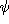we find the set of cocircular pairs of edges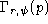. The magnitude of axial symmetry in the (p, r,) space is as follows: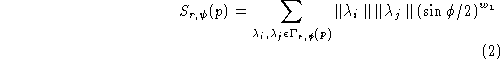where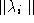and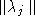are the edge intensities of the two co-circular edges andis the angle separating their normals.

Then, radial symmetry, I(p), is determined from the axial symmetry map as in Equationand Equation. Finally, the symmetry map undergoes Gaussian smoothing and local maxima are determined.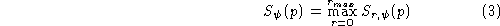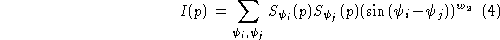We apply the symmetry transform twice for each image. First, on the interior of the table, we apply the general symmetry transform to find both dark and bright balls. Then, on the periphery of the table, we apply dark symmetry to find the consistently darker pockets.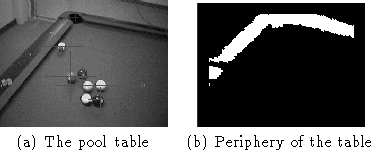Figure: Pool Table Periphery

Figureshows a pool table and the periphery where we might expect to find pockets (computed from the previous stage). Figure(a) displays the edge map of the interior of the table and (b) displays the edge map of the periphery of the table. Edge maps and phase maps (not shown) are computed using the Sobel operator. The edges due to green portions of the table are suppressed and the edge maps undergo some processing (non-maximal suppression and thresholding).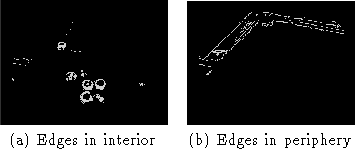Figure: Edge Detection

We then compute the symmetry transforms and obtain peaks of symmetry which have been overlaid on the table image in Figure. These peaks are triggered by balls and pockets (mostly) but there are some weak false alarm triggers. So, we wish to filter these candidate balls and pockets to reject false ones and also to label them.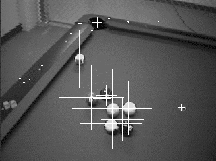Figure: Ball and Pocket CandidatesNext: Color Model Classification Up: Vision Processing Previous: Contour Computation

Tony Jebara
Wed Feb 18 18:52:15 EST 1998